Making 2D Hilbert Curve

Author: Zuguang Gu ( z.gu@dkfz.de )

Date: 2020-10-27

Package version: 1.20.0

Introduction

Hilbert curve is a type of space-filling curves that folds one dimensional axis into a two dimensional space, but still keeps the locality. It has advantages to visualize data with long axis in following two aspects:

1. greatly improve resolution of the visualization fron $$n$$ to $$\sqrt{n}$$;
2. easy to visualize clusters because generally data points in the axis will also be close in the 2D space.

This package aims to provide an easy and flexible way to visualize data through Hilbert curve. The implementation and example figures are based on following sources:

We first load the packages and set seed for random numbers.

library(HilbertCurve)
library(circlize)
set.seed(12345)

Following plots show Hilbert curves with level 2, 3, 4, 5: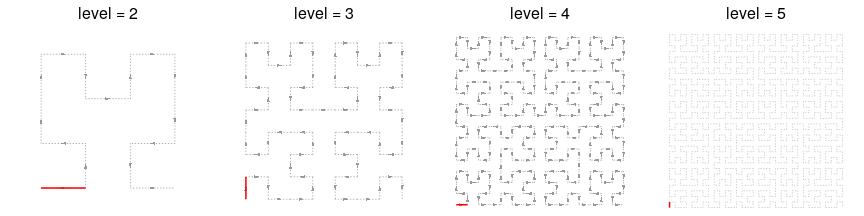As shown in the above plots, as level increases, the length of the curve becomes longer and the curve folds more densely. The number of segments (one segment is marked in red) on the Hilbert curve is 4^level - 1. If a Hilbert curve with level 11 is used to map to human chromosome 1, the resolution would be 249250621/4^11 (approximately 59bp per segment).

Locality

Hilbert curve folds one-dimensional axis into a two-dimensional space while still preserves the locality of the data points. We visualize this attribute with a Hilbert curve with level 5. In following animation, the point moves with its natural order on the axis.

for(i in 1:1024) {
hc = HilbertCurve(1, 1024, level = 5, reference = TRUE, arrow = FALSE)
hc_points(hc, x1 = i, np = NULL, pch = 16, size = unit(2, "mm"))
}

Next, we calculate the pairwise distance between data points in the 2D space and visualize it as heatmap. The numbers on x-axis (top) and y-axis (left) illustrate the position of data points in the original 1D axis.

library(HilbertVis)
pos = HilbertVis::hilbertCurve(5)
mat = as.matrix(dist(pos))
library(ComplexHeatmap)

ht = Heatmap(mat, name = "dist", cluster_rows = FALSE, cluster_columns = FALSE,
show_row_names = FALSE, show_column_names = FALSE,
heatmap_legend_param = list(title = "euclidean_dist"))
draw(ht, padding = unit(c(5, 5, 5, 2), "mm"))
decorate_heatmap_body("dist", {
grid.segments(c(0.25, 0.5, 0.75, 0, 0, 0), c(0, 0, 0, 0.25, 0.5, 0.75),
c(0.25, 0.5, 0.75, 1, 1, 1), c(1, 1, 1, 0.25, 0.5, 0.75), gp = gpar(lty = 2))
grid.text(rev(c(256, 512, 768, 1024)), 0, c(0, 256, 512, 768)/1024, just = "bottom",
rot = 90, gp = gpar(fontsize = 10))
grid.text(c(1, 256, 512, 768, 1024), c(1, 256, 512, 768, 1024)/1024, 1, just = "bottom",
gp = gpar(fontsize = 10))
})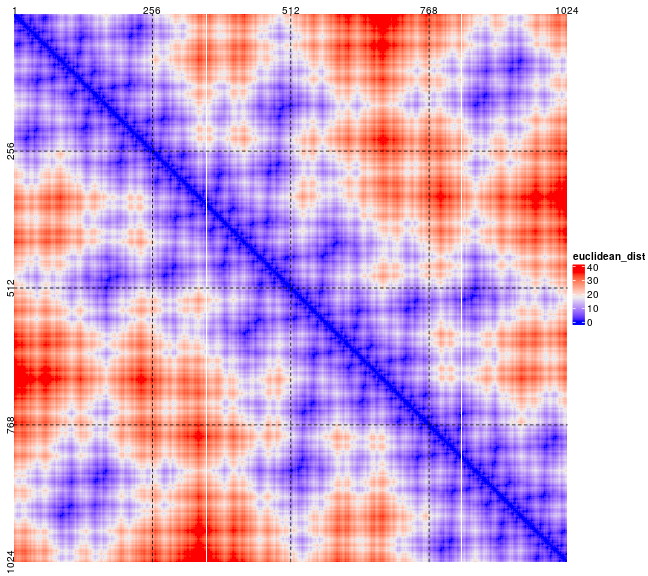Basically, from the heatmap, data points which are close in the 1D axis are still close in the 2D space (illustrated in the areas around diagonals).

Also there are some data points in (e.g, 1 ~ 256) which are close to data points in (768 ~ 1024)(bottom left or top right area in the heatmap), but it is only due to that the curve folds back. This exception can be easily distinguished by adding assistant grid lines or if users are clear with the structure of the curve.

Basic settings

The HilbertCurve package provides a rather simple way to display the data in the form of Hilbert curve. It hides all the technical parts and users only need to think that they are adding graphics on a novel axis based on specifying positions.

Generally, customizing a Hilbert curve follows following steps:

hc = HilbertCurve(...)    # initialize the curve

HilbertCurve() is a constructor function and initializes the Hilbert curve. Following example means initializing a Hilbert curve with level 4 which maps data ranging from 1 to 100. The function returns a HilbertCurve class instance and it can be used to add more graphics later.

reference argument here is only used to show the structure of the curve.

hc = HilbertCurve(1, 100, level = 4, reference = TRUE)

By default, the Hilbert curve starts from the bottom left corner. The start position can be specifyed by start_from option and the orientation of the first segment can be set by first_seg: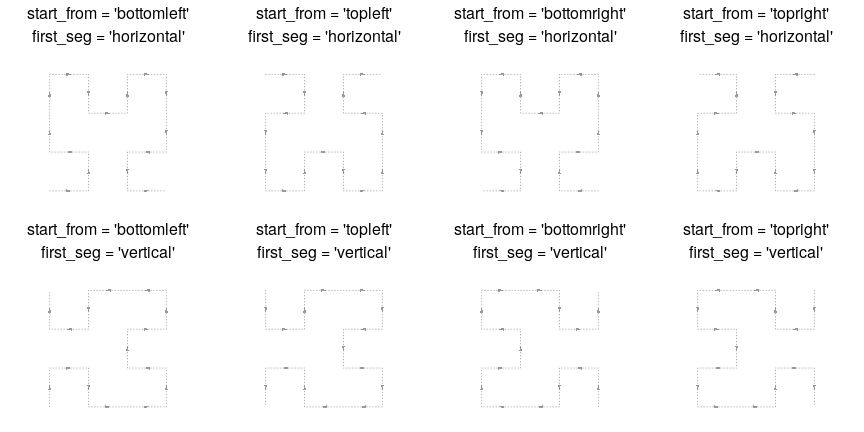The curve can be though as a folded axis. When the coordinate for this folded axis is initialized, low-level graphics can be added by specifying the positions.

There are several ways to specify the “positions” of the data points. The most common way is to construct a IRanges object:

library(IRanges)
x = sort(sample(100, 20))
s = x[1:10*2 - 1]
e = x[1:10*2]
ir = IRanges(s, e)
ir
## IRanges object with 10 ranges and 0 metadata columns:
##            start       end     width
##        <integer> <integer> <integer>
##             2        10         9
##            14        24        11
##            32        38         7
##            39        40         2
##            51        58         8
##            75        80         6
##            81        86         6
##            87        88         2
##            90        92         3
##           93        96         4

Here ir contains intervals which are composed by integers. In later sections, you will see it is also possible to specify positions with numeric values and very large values.

Points

There are two modes for adding points. Normally, intervals are always long in the curve and can not be sufficiently represented by single points, thus, by default, a list of e.g. circles compose the intervals.

hc = HilbertCurve(1, 100, level = 4, reference = TRUE)
hc_points(hc, ir)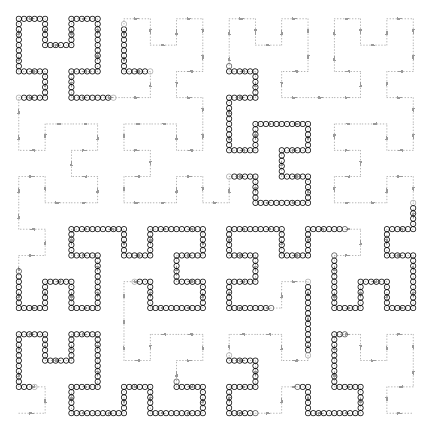The number of circles used to represent the intervals can be controlled by np (number of points per segment). np controls number of tiny segments that split every Hilbert curve segment (e.g. the first horizontal segment in the left bottom in the curve). Following plot is under np = 3.

hc = HilbertCurve(1, 16, level = 2, reference = TRUE, title = "np = 3")
hc_points(hc, x1 = 1, x2 = 2, np = 3)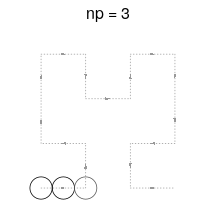Graphic parameters can be set by gp. Note under this mode, the size of points can only be controlled by np argument. To make it more interesting, you can choose different shapes for the points. There are some pre-defined shapes that you can choose from: “circle”, “square”, “triangle”, “hexagon”, “star”.

hc = HilbertCurve(1, 100, level = 4, reference = TRUE)
hc_points(hc, ir, np = 3, gp = gpar(fill = rand_color(length(ir))),
shape = sample(c("circle", "square", "triangle", "hexagon", "star"), length(ir), replace = TRUE))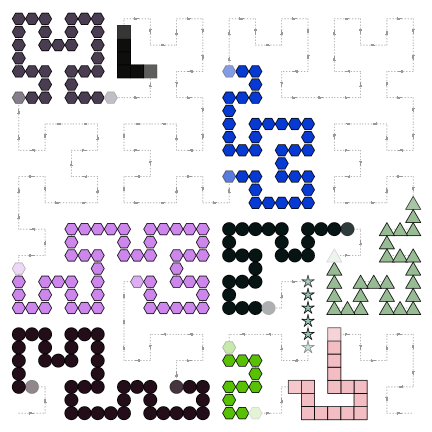In above figure, you may notice for some points, the color is more light in the ends. This is because the segments represented by these light circles are not fully covered by user's input intervals, thus, averaging is applied here. E.g. if only the half of the segment represented by the circle is covered by user's interval and the color for this circle is set to red, then after the averaging, the color would be semi-red (#FF8080, an average between red and white). Averaging is very important when you visualize the genome with zooming. You can find more detailed explanation in the Averaging section.

If np is set to 1 or NULL, there will be second mode for adding points that the points are plotted at the center of every interval in ir. In this case, size argument is used to control the size of the points. This mode is useful if you have a lot of small intervals.

hc = HilbertCurve(1, 100, level = 4, reference = TRUE)
hc_points(hc, ir, np = NULL, size = unit(runif(length(ir)), "cm"), pch = 16)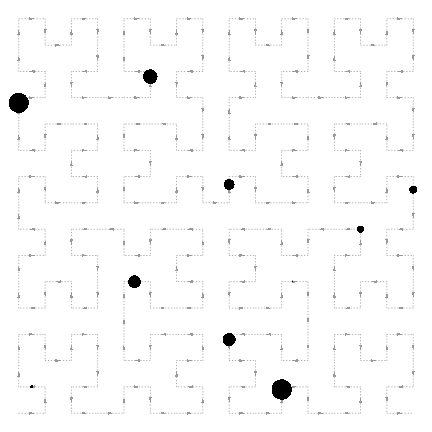Segments

Adding segments is quite straightforward. You can assign lwd to a large value to simulate long and twisted rectangles.

hc = HilbertCurve(1, 100, level = 4, reference = TRUE)
hc_segments(hc, ir, gp = gpar(lwd = 5))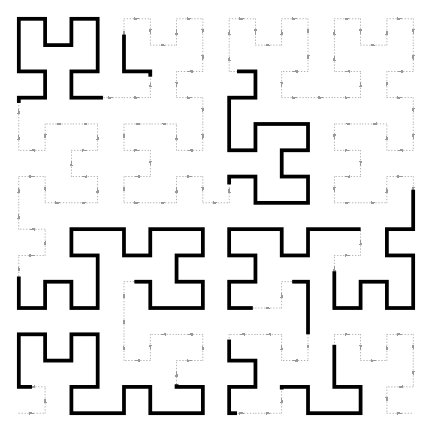Rectangles

hc_rect() fills intervals by a list of squares. The squares always locate at the turning points of the Hilbert curve and the width/height cannot be modified and it is automatically adjusted according to the level of the curve.

There is a difference from hc_points() with setting shape to square. The number of squares per segment is always 2 for hc_rect() while it can be any number for hc_points(). When the interval is long enough, hc_rect() can completely fill the surrounding areas.

hc = HilbertCurve(1, 100, level = 4, reference = TRUE)
hc_rect(hc, ir, gp = gpar(fill = "#FF000080"))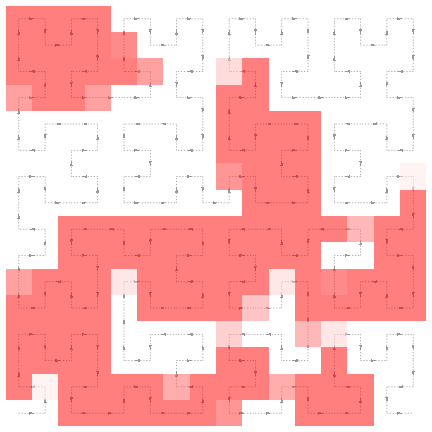As you can see some rectangles are not full red as well. It is also because of averaging. See Averaging sections for explanation.

Polygons

Basically polygons are quite similar to the rectangles. The major difference is 1. borders can be set for irregular polygons; 2. there is only single color in one polygon while for rectangles, the color can change in the ends of the interval if the corresponding segment is not completely covered by the input interval.

hc = HilbertCurve(1, 100, level = 4, reference = TRUE)
hc_polygon(hc, ir, gp = gpar(fill = "red", col = "black"))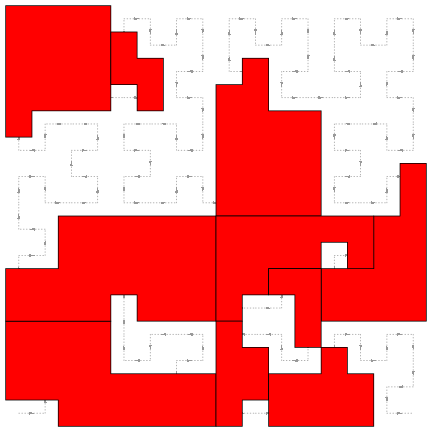Text

Text by hc_text() is always added at the center of each interval in ir. Additional settings such as just and rot can also be set.

hc = HilbertCurve(1, 100, level = 4, reference = TRUE)
labels = sample(letters, length(ir), replace = TRUE)
hc_text(hc, ir, labels = labels, gp = gpar(fontsize = width(ir)*2+5))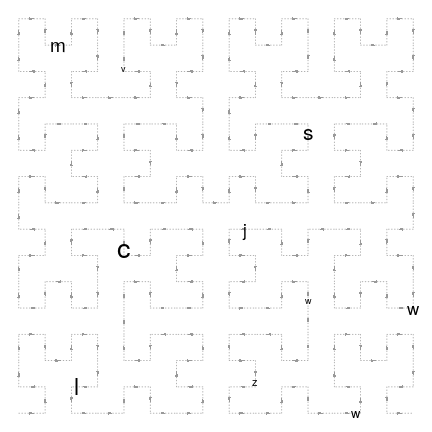When the intervals are long or when there are polygons representing the intervals, it is better to put text in the visual center of the polygons. For this, centered_by argument can be set to polygon. Compare postions of a and b in following plot:

hc = HilbertCurve(1, 100, level = 4, reference = TRUE)
hc_polygon(hc, ir)
hc_text(hc, ir, labels = "a")
hc_text(hc, ir, labels = "b", centered_by = "polygon")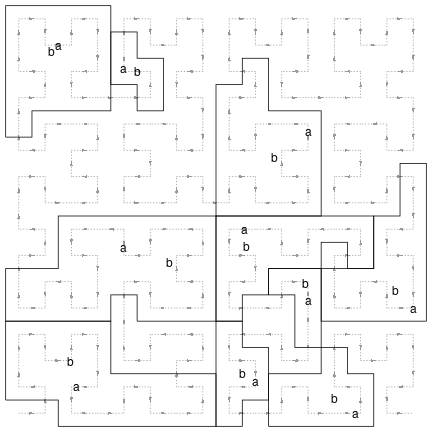This functionality is used to add chromosome names to the Hilbert curve.

Combine low-level functions

With combination of these basic low-level graphic functions, complicated graphics can be easily made:

hc = HilbertCurve(1, 100, level = 4)
hc_segments(hc, IRanges(1, 100))   # This is an other way to add background line
hc_rect(hc, ir, gp = gpar(fill = rand_color(length(ir), transparency = 0.8)))
hc_polygon(hc, ir[c(1,3,5)], gp = gpar(col = "red"))
hc_points(hc, ir, np = 3, gp = gpar(fill = rand_color(length(ir))),
shape = sample(c("circle", "square", "triangle", "hexagon", "star"), length(ir), replace = TRUE))
hc_text(hc, ir, labels = labels, gp = gpar(fontsize = width(ir)*2+5, col = "blue", font = 2))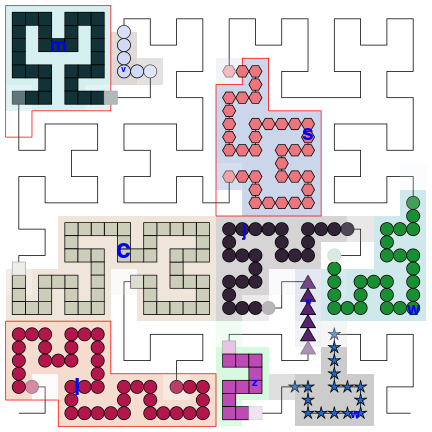Non-integer positions

It doesn't matter if your positions are integers or not. Internally, adjustment is automatically applied.

When positions are not integers, you can specify the positions by x1 and x2. All low-level graphical funtions accept x1 and x2.

hc = HilbertCurve(0.1, 0.8, level = 4, reference = TRUE)
hc_points(hc, x1 = c(0.15, 0.55), x2 = c(0.25, 0.65))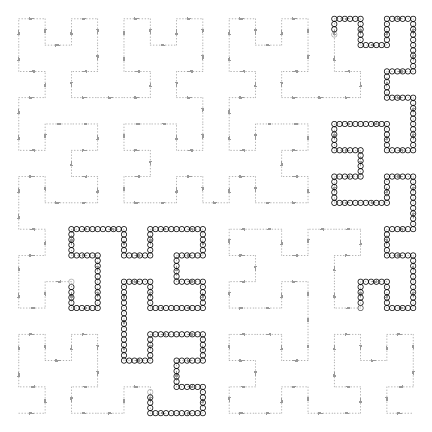This is also useful to specify the positions with huge integers which can not be handled by the IRanges class (e.g. the summation of total length of human chromosomes).

# code not run when generating the vignette
hc = HilbertCurve(1, 1000000000000, level = 4, reference = TRUE)
hc_points(hc, x1 = 400000000000, x2 = 600000000000)

Negative positions are allowed as well.

# code not run when generating the vignette
hc = HilbertCurve(-100, 100, level = 4, reference = TRUE)
hc_points(hc, x1 = -50, x2 = 50)

Pixel mode

The pixel mode can be thought as a high resolution version of hc_rect(). When the level is high (e.g. > 10), the whole 2D space will be almost completely filled by the curve and it is impossible to add or visualize e.g. points on the curve. In this case, the 'pixel' mode visualizes each tiny 'segment' as a pixel and maps values to colors. Internally, the whole plot is represented as an RGB matrix and every time a new layer is added to the plot, the RGB matrix will be updated according to the color overlay. When all the layers are added, normally a PNG figure is generated directly from the RGB matrix. So the Hilbert curve with level 11 will generate a PNG figure with 2048x2048 resolution. This is extremely useful for visualize genomic data. E.g. If we make a Hilbert curve for human chromosome 1 with level 11, then each pixel can represent 60bp (249250621/2048/2048) which is of very high resolution.

Under 'pixel' mode, every time a new layer is added, the image is added to the interactive device as a rastered image.

hc = HilbertCurve(1, 100, level = 9, mode = "pixel")
hc_layer(hc, ir)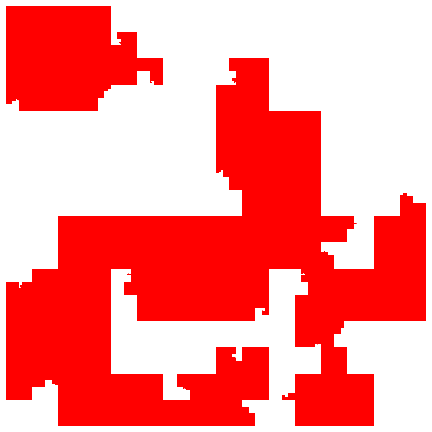Since color is graphic representation to values, there is only one graphic setting col. You can add more than one layers, and just remember to set transparent colors for the additional layers.

To map values to colors, users can try colorRamp2() function in circlize package to generate a color mapping function. Another advantage of using colorRamp2() is it can be used to generate the corresponding color legend.

col_fun = colorRamp2(c(0, 1), c("white", "red"))
x = seq(10, 960, length = 100)
x1 = x# start of each interval
x2 = x + 2    # end of each interval
value = runif(100)  # associated values
hc = HilbertCurve(1, 1000, level = 9, mode = "pixel", title = "pixel mode")
hc_layer(hc, x1 = x1, x2 = x2, mean_mode = "absolute", col = col_fun(value))
hc_layer(hc, x1 = 750, x2 = 850, col = "#00000010")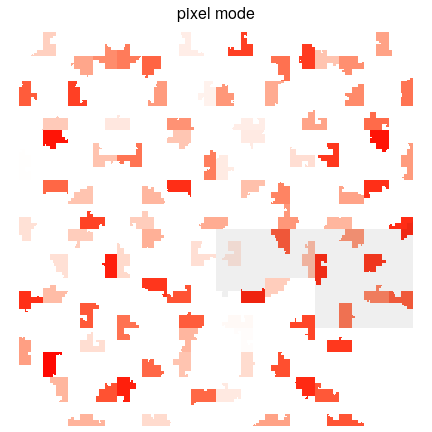Grid lines can be added to the plot for better distinguishing blocks in the Hilbert curve. The 2D space will be split into 2^(grid_line-1) rows and 2^(grid_line-1) columns.

hc = HilbertCurve(1, 1000, level = 9, mode = "pixel", title = "pixel mode")
hc_layer(hc, x1 = x1, x2 = x2, mean_mode = "absolute", col = col_fun(value), grid_line = 3)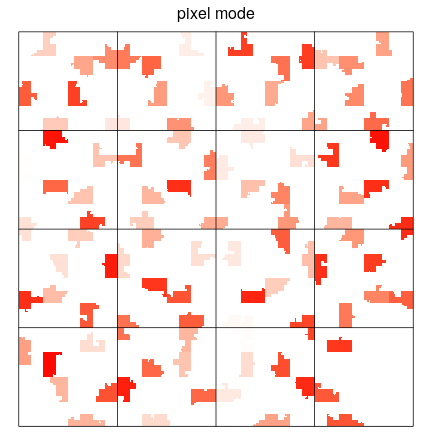Borders can be added by setting border to TRUE or a vector of colors to distinguish different intervals.

hc = HilbertCurve(1, 1000, level = 9, mode = "pixel", title = "pixel mode")
hc_layer(hc, x1 = x1, x2 = x2, mean_mode = "absolute", col = col_fun(value), border = TRUE)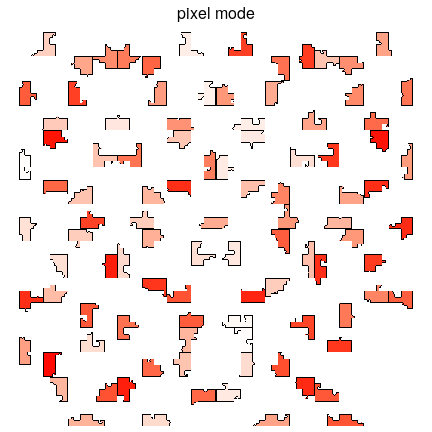Setting border argument in hc_layer directly modifies the RGB matrix. It adds one pixel border for each polygon and sometimes the border dispears when compressing the image. It is more suggested to add borders by hc_polygon():

hc = HilbertCurve(1, 1000, level = 9, mode = "pixel", title = "pixel mode")
hc_layer(hc, x1 = x1, x2 = x2, mean_mode = "absolute", col = col_fun(value))
hc_polygon(hc, x1 = x1, x2 = x2)